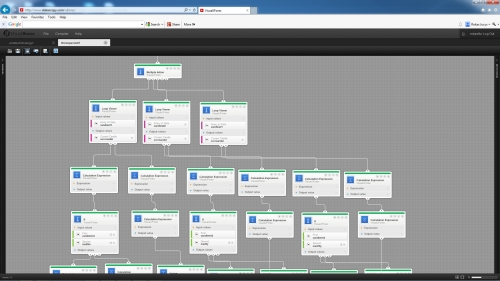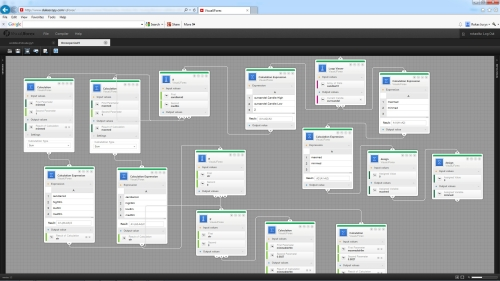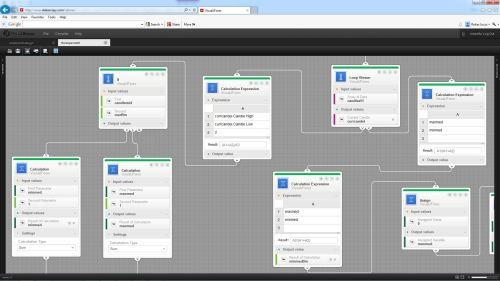1. Overview

In my previous publications of 3 period max/median/min strategy basic principles of strategy were covered and some trades based on outcome of mentioned strategy calculations were entered. In may last publication I covered automation of strategy need and described how to use visual jforex for calculating of part of variables needed for strategy input. In this publication I will cover possible algorithm for automated calculations of remaining variables required for strategy. This will include TimeMaxMedian, TimeMinMedian, MaxMedianStrength, MinMedianStrength for all required periods (10 year, 2 year and 9 months) automated calculation.
It should be noted that strategy is under development and is not suitablefor real trades.

2. Algorithm description

In order to calculate remaining variables needed for strategy input all previously created visual jforex logic for max, median and min values calculation will be used as basement. As in logic which was described during last month publication all arrays of data for all 3 period analysis were already prepared then developing this logic only usage of data and different kind of calculations were needed. Thus Multiple Action block 4th output was used for branch needed to performed calculations of remaining variables. This branch is connected with another Multiple Action block from which TimeMaxMedian, TimeMinMedian, MaxMedianStrength, MinMedianStrength calculations starts. This is depicted in picture below:In order to understand how this logic is organized analysis of variables for one period is needed. Calculations for other periods are done by the same principles only different arrays of data are used and parameters which participate in calculations are altered according to candles number in certain period.
How calculation are arranged it is shown in picture below:For deeper analysis I will split logic in to parts - first for calculation analysis of TimeMaxMedian, TimeMinMedian and second for calculation analysis of MaxMedianStrength, MinMedianStrength.

3. TimeMaxMedian and TimeMinMedian calculations description

Workflow for calculating of these variables is shown in picture below:Logic starts with analyzing of array of 1 day candles which are stored in automatically by system created variable candles51. For performing of this analysis Loop Viewer block is used. This block takes every 1 day candle from stored 1 day candles of 9 month period and activates 1st output. After this logic goes to Calculation Expression block and in this block average of candle is calculated. Further on logic passes to If block which compares if candle middle is higher or lower than 9 month period median. If it is higher temporary variable maxmed is increased by 1 point and if it is lower temporary variable minmed is increased by 1 point. These mathematical calculations are done by Calculation block means. So logic actually calculates number of candles which are higher than median and number of candles which is lower than median.
After all candles from 9 month daily candles array are analyzed, Loop Viewer block activates its second output. Here Calculation expression blocks are used in order from temporary variables calculate actual TimeMaxMedian and TimeMinMedian values. It is done by simple formula which divides number of candles higher than median by all candles in the array and number of candles lower than median by all candles in the array. After calculations are done temporary variables (which later will be used for calculations in other periods) are set to 0 by means of Assign blocks.
Thus calculations of mentioned parameters are done for 9 month period and later on the same logic is applied for calculations of these parameters for other periods.

4. MaxMedianStrength and MinMedianStrength calculations description

Workflow for calculating of these variables is shown in picture below:This workflow is continuation of TimeMaxMedian and TimeMinMedian calculation workflow. After each candle from candle array is taken for analysis and analyzed is it above or below median and calculation of temporary variable maxmed or minmed is performed flow continues with analysis if candle is high enough from median in order to increase MaxMedianStrength variable or low enough from median in order to increase MinMedianStrength variable.
This is performed with Calculation Expression block which subtracts from middle of the candle value half of difference of 9 months median and minimum points in case of MinMedianStrength calculation and half of a difference of 9 months period maximum and median points in case of MaxMedianStrength calculation. Later on comparison of calculated value with 0 is done and if distance from median is enough (result greater than 0) to variable which initial (start) value is 0.5 0.0027 points are added. If not variable is left intact.
Thus calculations of mentioned parameters are done for 9 month period and later on the same logic is applied for calculations of these parameters for other periods.

5. Outcome of logic

Thus by having this logic we can change instrument in one place (change default instrument in visual jforex variables area) run visual jforex workflow and all required parameters will be depicted. Later on these parameters could be put to excel sheet which calculates actual outcome of strategy, i.e. to open trade or not and if open in which direction and with what parameters. Just in order to test its performance lets see what outcome will be with GBP/CAD pair:So we can see that all required variables was calculated. But after putting variables in excel for actual outcome now trade signal was issued that means strategy is not sure in which direction rate will move. After analyzing few more pairs some trade signals were issued and based on this one test trade was entered ENTRY SELL USD/NOK at 6.169 STOP LOSS at 6.269 TAKE PROFIT at 6.009.6. Further steps

After automation of strategy is done I plan to test it more intensively. But actually there is one more point which I consider. Calculations are done in visual jforex but for for final outcome of the strategy excel is needed. I am considering possibility to put everything in visual jforex but some more time is needed for this as calculations are quite complex and I don't know if I succeed to put it in visual jforex platform. But this will be the topic of coming publications.
Translate to English Show original